# Gauss theorem

(diff) ← Older revision | Latest revision (diff) | Newer revision → (diff)

(teorema egregium)

The Gaussian curvature (the product of the principal curvatures) of a regular surface in Euclidean space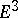remains unchanged when the surface is isometrically deformed. ( "Regularity" here means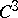-smooth immersion.) Gauss' theorem follows from the fact that the Gaussian curvatureof a surface at a point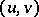can be expressed in terms of the coefficients of the first fundamental form of the surface,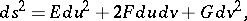and their first and second derivatives at that point. Such an expression foris called the Gauss equation, which may be written down in several forms . The Gauss equation simplifies in special coordinates. Thus, in isothermal coordinates (,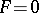):in semi-geodesic coordinates (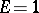,):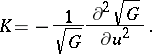The Gauss equation and the Peterson–Codazzi equations form the conditions for the integrability of the system to which the problem of the reconstruction of a surface from its first and second fundamental forms may be reduced. It follows from Gauss' theorem and from the Gauss–Bonnet theorem that the difference between the sum of the angles of a geodesic triangle on a regular surface andis equal to the oriented area of the spherical image of this triangle .

Gauss' theorem was established by C.F. Gauss  and it is the first and most important result in the study of the relations between the intrinsic and the extrinsic geometry of surfaces.

The following generalization of Gauss' theorem is valid ,  for a regular-dimensional, surface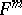in a Riemannian space,:(*)

where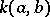,are the sectional curvatures of, respectively,and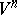in the two-dimensional direction defined by the tangent vectorstoat the point under consideration, and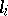is the second fundamental form ofwith respect to the-th normal of an orthonormal set of normals at this point. It follows from (*) that, for a hypersurface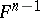in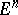, all even elementary symmetric functions of the principal curvatures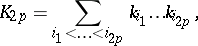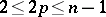, are defined by the first fundamental form of. In an even-dimensional space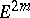,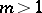, a hypersurface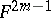is uniquely defined by its first fundamental form and by the Gauss–Kronecker curvatureon the condition that the latter is non-zero .

For large classes of two-dimensional irregular surfaces init is possible to define an "external curvatureexternal curvature" as a Borel measure connected with the spherical mapping and an "intrinsic curvatureintrinsic curvature" as a measure connected with the difference between the sum of the angles of a triangle and. A generalization of Gauss' theorem is the statement that the external and the internal curvatures coincide. Such a generalization of Gauss' theorem was obtained for general convex surfaces  and for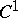-smooth surfaces of bounded external curvature .

How to Cite This Entry:
Gauss theorem. Encyclopedia of Mathematics. URL: http://encyclopediaofmath.org/index.php?title=Gauss_theorem&oldid=15763
This article was adapted from an original article by Yu.D. Burago (originator), which appeared in Encyclopedia of Mathematics - ISBN 1402006098. See original article